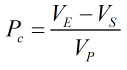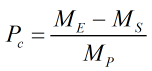# C132 – Sampler Control Pulse Check

## Description

This calculates the number of pulses expected for a volume or mass at a given pulse significance factor.

Kelton calculation reference C132

KIMS calculation reference K162

## Options

### Sampler type

• Volume based

This option is chosen to perform the volume based calculation.

• Mass based

This option is chosen to perform the mass based calculation.

## Calculation

### Corrected something

When using the volume based method the number of pulses is calculated by:Where VE = Total volume at the end VS = Total volume at the start VP Pulse significance factor

When using the mass based method the number of pulses is calculated by:Where ME = Total mass at the end MS = Total mass at the start MP Pulse significance factor

Back